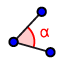# Transversals

## Answer the questions below the diagram.

Use the green slider at the bottom right to change the measure (m) of BAC. Measure angles by opening the diagram with the GeoGebra app (from the upper right menu); then, use this toolto select three points: two point on either side of the vertex of the angle measured. The points must be chosen in the counterclockwise direction from the point on one side to the vertex to the point on the other side of the angle.

1. If the measure ofBAC is 41°, what is the mKAM?

Check all that apply

2. Which angle is vertical to FCA?

Check all that apply

3. If the mBAC is 63°, what is the mLCE?

Check all that apply

4. Which is the corresponding angle to LCP?

Check all that apply

5. If the mBAC is 145°, what is the measure of CAN

Check all that apply

6. If the mKAN is 110 degrees, what is the angle measure (in degrees) of NCA

Check all that apply